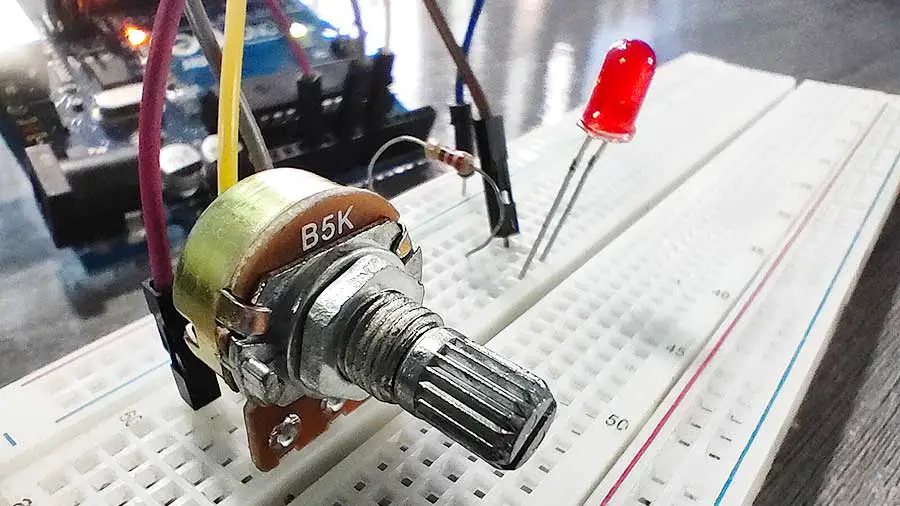Potentiometers are one of the commonly used electronic parts. They are used to control sound volume, the brightness of a light source, changing frequencies on a radio, and so many other things. There are many types of potentiometers but in this article, we will be using the rotary potentiometer.

A rotary potentiometer is a simple knob that you can turn clockwise or counterclockwise. By turning the potentiometer in either direction, you are changing its resistance value. This variable resistance can then be read by an Arduino board as an analog value.

When the knob is turned all the way in one direction, there will be 0 volts going to the Arduino analog pin. In this case, the Arduino will read a 0. If it is turned all the way in the opposite direction, there will be 5 volts going to the Arduino analog pin. In this case, the Arduino will read 1023.The potentiometer has 3 terminal pins. The center pin goes to an analog pin while the outer pins go to 5V and GND pins on the Arduino board.  The outer pins can be interchanged.

Here are two Arduino projects from the Learn Arduino Intro app to get you started with the rotary potentiometer.

# PROJECT 1: Potentiometer Value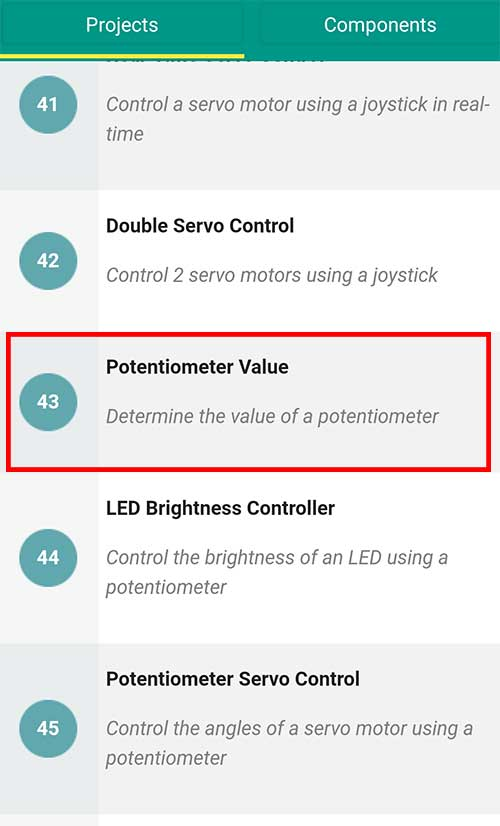This project simply displays the value we read from a potentiometer and displays it on the Serial Monitor.

Materials:

• 1 Rotary Potentiometer
• 1 Arduino Microcontroller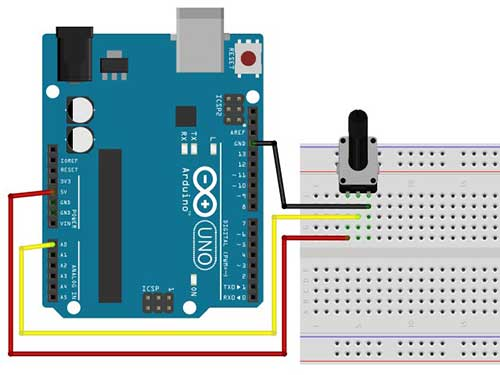Arduino Sketch:

``````

//Read the value of a potentiometer

int pot_pin=A0; // potentiometer is connected to analog pin A0
int val=0;

void setup()
{
//initialize communication at 9600 bits per second
Serial.begin(9600);
}

void loop() {

//print the value on the serial monitor
//Go to Tools->Serial Monitor to see the values
Serial.println(val);
delay(100);
}
``````

The sketch is pretty much straightforward. The important part here is the analogRead function. The analogRead() reads the value coming from the specified analog pin. Since it is analog, we expect a reading from 0 to 1023. The value is then saved into the val variable and printed on the Serial Monitor by writing this line:

``    Serial.println(val);``

# PROJECT 2: LED Brightness Controller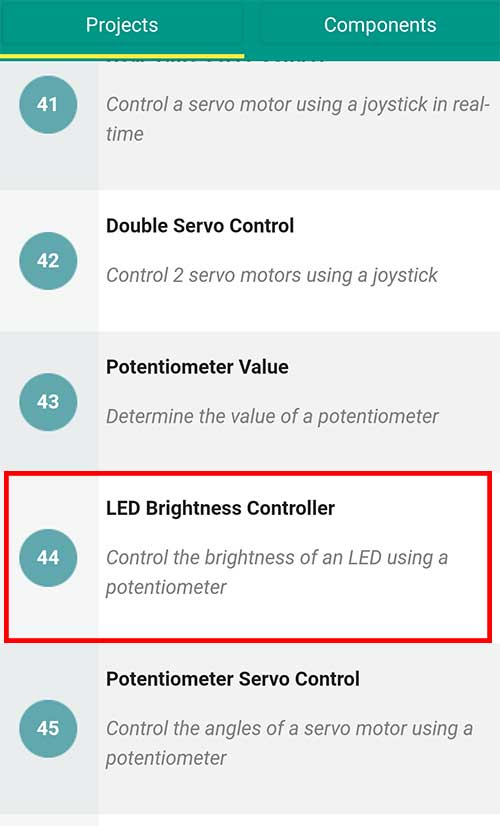In this project, we will control the brightness of an LED using the potentiometer.

Materials:

• 1 Rotary Potentiometer
• 1 LED
• 1 220-ohm Resistor (red-red-brown)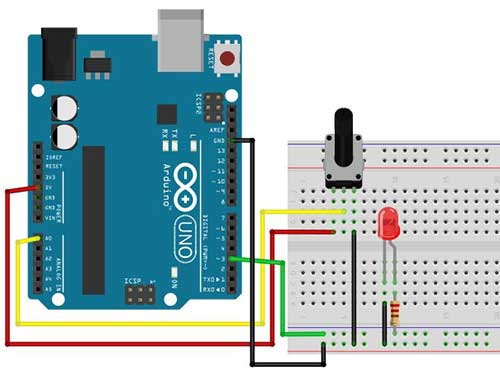Arduino Sketch:

``````  //use potentiometer to fade an LED
//LED should be connected to PWM pins (3,5,6,9,10,11)

int pot_pin=A0; // potentiometer is connected to analog pin A0
int val=0;
int ledPin = 3;    // LED connected to digital pin 3

void setup() {
// initialize the digital pin as an output.
pinMode(ledPin, OUTPUT);
}

void loop() {

// read the value from the potentiometer and save to val variable
// convert val reading to pwm value (0-255)
val = map(val, 0, 1023, 0, 255);

analogWrite(ledPin, val);
// wait for 30 milliseconds before the next potentiometer reading
delay(30);

}``````

Just like in the previous project, we read the value of the potentiometer by writing:

``val = analogRead(pot_pin); ``

In order to change the brightness of an LED, you have to connect it to a PWM pin. To know more about PWM and the fading LED effect, you may read this article.

The PMW pins on an Arduino Uno board are 3,5,6,9,10, and 11. We can assign a value to a PWM pin ranging from 0 to 255.

The value of the potentiometer is stored in the variable val. This value is what we will be using as our PWM value. However, there is a slight problem. The value coming from the potentiometer ranges from 0 to 1023, while PWM values should only range from 0 to 255. Therefore, we need a way to modify the potentiometer’s value so that it won’t exceed 255.

To do this we need to use the map() function. The map() function re-maps a number from one range to another. That is, a value of fromLow would get mapped to toLow, a value of fromHigh to toHigh, values in-between to values in-between, etc.

This is the syntax of the map()

``map(value, fromLow, fromHigh, toLow, toHigh)``

We will also assign the new mapped value into the same val variable like this:

`` val = map(val, 0, 1023, 0, 255); ``

Therefore, if the potentiometer’s value is 0 (fromLow), the mapped value becomes 0 (toLOW). If the value is 1023 (fromHigh), the mapped value becomes 255 (toHigh).

Now that we have mapped the val variable with a range from 0 to 255, we can use it in our analogWrite function.

`` analogWrite(ledPin, val);``

A delay of 30 milliseconds is added to avoid too much flickering on the LED.